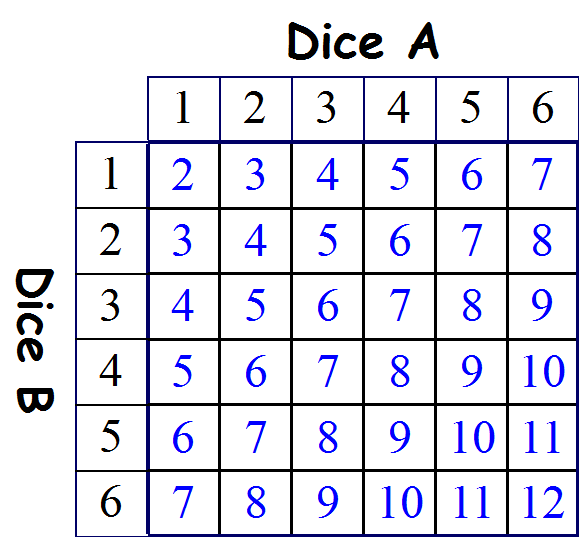# Handling Data - Probability 2

## What is the probability of throwing a total of 1?

It is impossible because by throwing two dice, the lowest score we could ever achieve would be 2 - a '1' on the first dice and a '1' on second dice. So the probability is 0

## What is the probability of throwing a total of 12?

We would have to throw a 6 with both dice. How many outcomes are there?

Lets label the two dice, dice A and dice B. Dice A could land on 1,2,3,4,5 or 6, and
If dice A lands on 1, what outcomes are there for dice B? It could land on 1,2,3,4,5 or 6
If dice A lands on 2, what outcomes are there for dice B? It could land on 1,2,3,4,5 or 6
If dice A lands on 3, what outcomes are there for dice B? It could land on 1,2,3,4,5 or 6
If dice A lands on 4, what outcomes are there for dice B? It could land on 1,2,3,4,5 or 6
If dice A lands on 5, what outcomes are there for dice B? It could land on 1,2,3,4,5 or 6
If dice A lands on 6, what outcomes are there for dice B? It could land on 1,2,3,4,5 or 6

So there are 6 different combinations of 6 .... 6 outcomes for each position that dice A could land on ....... 6 x 6 = 36 possible outcomes.

This is getting complicated - lets make it easier to understand with a table.This table shows all the possible totals by adding all the possible combinations from Dice A and Dice B. As we deduced before, there are 36 different combinations.

So by looking at the table there are 36 possible outcomes, how many of those outcomes result in a twelve, just one - 1 out of 36.

As a fraction 1/36 ... As a decimal 1 ÷ 36 = 0.028 ... As a percentage 0.028 x 100 = 2.8%

## What is the probability of throwing a total of 2?

by looking at the table there are 36 possible outcomes, how many of those outcomes result in a two, just one - 1 out of 36.

As a fraction 1/36 ... As a decimal 1 ÷ 36 = 0.028 ... As a percentage 0.028 x 100 = 2.8%

## What is the probability of throwing a total of 3?

by looking at the table there are 36 possible outcomes, how many of those outcomes result in a three, just two - 2 out of 36.

As a fraction 2/36 which can be simplified to 1/18 ... As a decimal 1 ÷ 18 = 0.055
... As a percentage 0.055 x 100 = 5.5%

## What is the probability of throwing a total of 7?

by looking at the table there are 36 possible outcomes, how many of those outcomes result in a seven, there are six - 6 out of 36.

As a fraction 6/36 which can be simplified to 1/6 ... As a decimal 1 ÷ 6 = 0.166
... As a percentage 0.166 x 100 = 16.6%

### By using a table, we have made it easier to understand the data, but we are still doing the same thing - Working out all the possible outcomes.

Play in Full Screen